## Solution to 1986 Problem 46

 The relevant formula is the Stefan-Boltzmann formula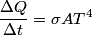\begin{align*}\frac{\Delta Q}{\Delta t} = \sigma A T^4\end{align*}Here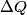$\Delta Q$/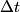$\Delta t$ is the rate at which energy is radiated from the blackbody,$\sigma$ is the Stefan-Boltzmann constant,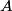$A$ is the area of the blackbody, and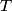$T$ is the temperature of the blackbody in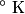$^{\circ} \mbox{ K}$. So, doubling$T$ while holding everything else constant increases the power radiated by the blackbody by a factor of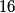$16$. Thus, answer (E) is correct.